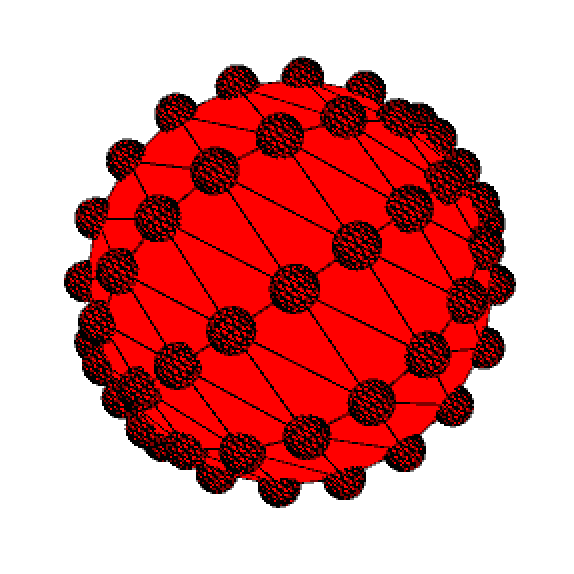#### Howdy, Stranger!

We are about to switch to a new forum software. Until then we have removed the registration on this forum.

# Drawing points for each vertex on a rotating 3D sphere

edited March 2017

I'm trying to draw each vertex of a rotating 3D sphere as points in 2D coordinate screen space. Here's my attempt.

``````    PShape my_sphere;
ArrayList<PVector> vertices = new ArrayList<PVector>();

void setup(){
size(800, 600, P3D);
frameRate(60);
smooth();
sphereDetail(10);
fill(255,0,0);
my_sphere = createShape(SPHERE, 100);
}

void draw_sphere(){
pushMatrix();
translate(width/2, height/2);
rotateZ(millis() * 0.0001 * TWO_PI);
//rotateY(millis() * 0.0001 * TWO_PI);
shape(my_sphere);
my_sphere.setVisible(false);
popMatrix();
}

void draw_points(){
getVertices(my_sphere, vertices);
for(int i=0; i < vertices.size(); i++){
PVector vertex = vertices.get(i);
float x = vertex.x;
float y = vertex.y;
float z = vertex.z;

float s_x = screenX(x, y, z);
float s_y = screenY(x, y, z);

point(s_x+(width/2), s_y+(height/2));
}
}

void draw(){
background(255,255,255);
draw_sphere();
draw_points();
vertices.clear();
}

void getVertices(PShape shape, ArrayList<PVector> vertices){
for(int i = 0 ; i < shape.getVertexCount(); i++){
PVector vertex = shape.getVertex(i);
vertices.add(vertex);
}
}
``````

The issue I'm seeing is that as the sphere rotates (which I've hidden) the points aren't updating to match. Why? I've tried lots of things and can't seem to figure it out. Help would be appreciated.

Tagged:

## Answers

• @gvdb -- You have several related issues with this code.

1. draw() calls draw_sphere() and draw_points() -- your rotation happens in draw_sphere(). So your points don't rotate! Put your rotation in draw, around both the sphere and the point. Now both the sphere and the points rotate!

2. draw_points() is doing a lot of unnecessary stuff with offsetting. Don't do that. Just draw each point at x,y,z -- or translate before drawing each small sphere. If you want to shift the whole point set, do it as a single translate or rotate before calling draw_points(), don't do it inside draw_points().

Here is a simple example based on your code:

``````PShape my_sphere;
ArrayList<PVector> vertices = new ArrayList<PVector>();

void setup(){
size(800, 600, P3D);
frameRate(60);
smooth();
sphereDetail(10);
fill(255,0,0);
my_sphere = createShape(SPHERE, 100);
}

void draw(){
background(255,255,255);
pushMatrix();
translate(width/2, height/2);
rotateZ(millis() * 0.0001 * TWO_PI);
rotateY(millis() * 0.0001 * TWO_PI);
draw_sphere();
draw_points();
vertices.clear();
popMatrix();
}

void draw_sphere(){
shape(my_sphere);
my_sphere.setVisible(true);
}

void draw_points(){
getVertices(my_sphere, vertices);
for(int i=0; i < vertices.size(); i++){
PVector vertex = vertices.get(i);
float x = vertex.x;
float y = vertex.y;
float z = vertex.z;
pushMatrix();
translate(x, y, z);
sphere(10);
popMatrix();
}
}

void getVertices(PShape shape, ArrayList<PVector> vertices){
for(int i = 0 ; i < shape.getVertexCount(); i++){
PVector vertex = shape.getVertex(i);
vertices.add(vertex);
}
}
``````Other recent code on the forum relating to sphere rotation and points-on-a-sphere:

Sign In or Register to comment.